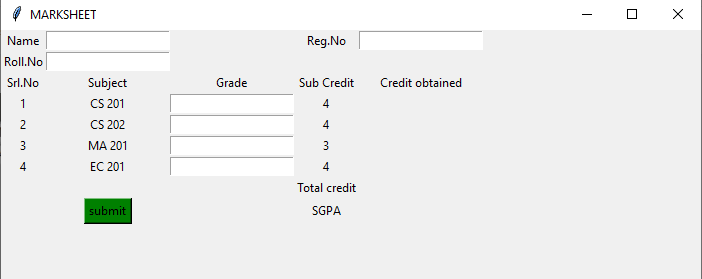# Python | Create a GUI Marksheet using Tkinter

• Difficulty Level : Medium
• Last Updated : 29 May, 2021

Create a python GUI mark sheet. Where credits of each subject are given, enter the grades obtained in each subject and click on Submit. The credits per subject, the total credits as well as the SGPA are displayed after being calculated automatically. Use Tkinter to create the GUI interface.

Refer the below articles to get the idea about basics of tkinter and Python.

Python offers multiple options for developing a GUI (Graphical User Interface). Out of all the GUI methods, Tkinter is the most commonly used method. It is a standard Python interface to the Tk GUI toolkit shipped with Python. Python with Tkinter outputs the fastest and easiest way to create GUI applications. Creating a GUI using Tkinter is an easy task.

To create a Tkinter:

• Importing the module – Tkinter
• Create the main window (container)
• Add any number of widgets to the main window
• Apply the event Trigger on the widgets.

This is how the GUI would look:Let’s create a GUI-based simple mark sheet using the Python Tkinter module, which can create a mark sheet based on the marks entered per subject.

Below is the implementation :

## Python3

 `# Python program to create a``# GUI mark sheet using tkinter`  `# Import tkinter as tk``import` `tkinter as tk`  `# creating a new tkinter window``master ``=` `tk.Tk()` `# assigning a title``master.title(``"MARKSHEET"``)` `# specifying geometry for window size``master.geometry(``"700x250"``)`  `# declaring objects for entering data``e1 ``=` `tk.Entry(master)``e2 ``=` `tk.Entry(master)``e3 ``=` `tk.Entry(master)``e4 ``=` `tk.Entry(master)``e5 ``=` `tk.Entry(master)``e6 ``=` `tk.Entry(master)``e7 ``=` `tk.Entry(master)`   `# function to display the total subject``# credits total credits and SGPA according``# to grades entered``def` `display():``    ` `    ``# Variable to store total marks``    ``tot``=``0``    ` `    ``# 10*number of subject credits``    ``# give total credits for grade A``    ``if` `e4.get() ``=``=` `"A"``:``        ` `        ``# grid method is used for placing``        ``# the widgets at respective positions``        ``# in table like structure .``        ``tk.Label(master, text ``=``"40"``).grid(row``=``3``, column``=``4``)``        ``tot ``+``=` `40``        ` `    ``# 9*number of subject credits give``    ``# total credits for grade B``    ``if` `e4.get() ``=``=` `"B"``:``        ``tk.Label(master, text ``=``"36"``).grid(row``=``3``, column``=``4``)``        ``tot ``+``=` `36``        ` `    ``# 8*number of subject credits give``    ``# total credits for grade C``    ``if` `e4.get() ``=``=` `"C"``:``        ``tk.Label(master, text ``=``"32"``).grid(row``=``3``, column``=``4``)``        ``tot ``+``=` `32``        ` `    ``# 7*number of subject credits``    ``# give total credits for grade D   ``    ``if` `e4.get() ``=``=` `"D"``:``        ``tk.Label(master, text ``=``"28"``).grid(row``=``3``, column``=``4``)``        ``tot ``+``=` `28``        ` `    ``# 6*number of subject credits give``    ``# total credits for grade P   ``    ``if` `e4.get() ``=``=` `"P"``:``        ``tk.Label(master, text ``=``"24"``).grid(row``=``3``, column``=``4``)``        ``tot ``+``=` `24``        ` `    ``# 0*number of subject credits give``    ``# total credits for grade F   ``    ``if` `e4.get() ``=``=` `"F"``:``        ``tk.Label(master, text ``=``"0"``).grid(row``=``3``, column``=``4``)``        ``tot ``+``=` `0`` ` ` ` `    ``# Similarly doing with other objects``    ``if` `e5.get() ``=``=` `"A"``:``        ``tk.Label(master, text ``=``"40"``).grid(row``=``4``, column``=``4``)``        ``tot ``+``=` `40``    ``if` `e5.get() ``=``=` `"B"``:``        ``tk.Label(master, text ``=``"36"``).grid(row``=``4``, column``=``4``)``        ``tot ``+``=` `36``    ``if` `e5.get() ``=``=` `"C"``:``        ``tk.Label(master, text ``=``"32"``).grid(row``=``4``, column``=``4``)``        ``tot ``+``=` `32``    ``if` `e5.get() ``=``=` `"D"``:``        ``tk.Label(master, text ``=``"28"``).grid(row``=``4``, column``=``4``)``        ``tot ``+``=` `28``    ``if` `e5.get() ``=``=` `"P"``:``        ``tk.Label(master, text ``=``"28"``).grid(row``=``4``, column``=``4``)``        ``tot ``+``=` `24``    ``if` `e5.get() ``=``=` `"F"``:``        ``tk.Label(master, text ``=``"0"``).grid(row``=``4``, column``=``4``)``        ``tot ``+``=` `0``     ` `     ` ` ` `    ``if` `e6.get() ``=``=` `"A"``:``        ``tk.Label(master, text ``=``"30"``).grid(row``=``5``, column``=``4``)``        ``tot ``+``=` `30``    ``if` `e6.get() ``=``=` `"B"``:``        ``tk.Label(master, text ``=``"27"``).grid(row``=``5``, column``=``4``)``        ``tot ``+``=` `27``    ``if` `e6.get() ``=``=` `"C"``:``        ``tk.Label(master, text ``=``"24"``).grid(row``=``5``, column``=``4``)``        ``tot ``+``=` `24``    ``if` `e6.get() ``=``=` `"D"``:``        ``tk.Label(master, text ``=``"21"``).grid(row``=``5``, column``=``4``)``        ``tot ``+``=` `21``    ``if` `e6.get() ``=``=` `"P"``:``        ``tk.Label(master, text ``=``"28"``).grid(row``=``5``, column``=``4``)``        ``tot ``+``=` `24``    ``if` `e6.get() ``=``=` `"F"``:``        ``tk.Label(master, text ``=``"0"``).grid(row``=``5``, column``=``4``)``        ``tot ``+``=` `0`` ` ` ` ` ` ` ` `    ``if` `e7.get() ``=``=` `"A"``:``        ``tk.Label(master, text ``=``"40"``).grid(row``=``6``, column``=``4``)``        ``tot ``+``=` `40``    ``if` `e7.get() ``=``=` `"B"``:``        ``tk.Label(master, text ``=``"36"``).grid(row``=``6``, column``=``4``)``        ``tot ``+``=` `36``    ``if` `e7.get() ``=``=` `"C"``:``        ``tk.Label(master, text ``=``"32"``).grid(row``=``6``, column``=``4``)``        ``tot ``+``=` `32``    ``if` `e7.get() ``=``=` `"D"``:``        ``tk.Label(master, text ``=``"28"``).grid(row``=``6``, column``=``4``)``        ``tot ``+``=` `28``    ``if` `e7.get() ``=``=` `"P"``:``        ``tk.Label(master, text ``=``"28"``).grid(row``=``6``, column``=``4``)``        ``tot ``+``=` `24``    ``if` `e7.get() ``=``=` `"F"``:``        ``tk.Label(master, text ``=``"0"``).grid(row``=``6``, column``=``4``)``        ``tot ``+``=` `0`` ` ` ` `    ``# to display total credits``    ``tk.Label(master, text``=``str``(tot)).grid(row``=``7``, column``=``4``)``    ` `    ``# to display SGPA``    ``tk.Label(master, text``=``str``(tot``/``15``)).grid(row``=``8``, column``=``4``)` `    ` `# end of display function` `# label to enter name``tk.Label(master, text``=``"Name"``).grid(row``=``0``, column``=``0``)` `# label for registration number``tk.Label(master, text``=``"Reg.No"``).grid(row``=``0``, column``=``3``)` `# label for roll Number``tk.Label(master, text``=``"Roll.No"``).grid(row``=``1``, column``=``0``)` `# labels for serial numbers``tk.Label(master, text``=``"Srl.No"``).grid(row``=``2``, column``=``0``)``tk.Label(master, text``=``"1"``).grid(row``=``3``, column``=``0``)``tk.Label(master, text``=``"2"``).grid(row``=``4``, column``=``0``)``tk.Label(master, text``=``"3"``).grid(row``=``5``, column``=``0``)``tk.Label(master, text``=``"4"``).grid(row``=``6``, column``=``0``)`  `# labels for subject codes``tk.Label(master, text``=``"Subject"``).grid(row``=``2``, column``=``1``)``tk.Label(master, text``=``"CS 201"``).grid(row``=``3``, column``=``1``)``tk.Label(master, text``=``"CS 202"``).grid(row``=``4``, column``=``1``)``tk.Label(master, text``=``"MA 201"``).grid(row``=``5``, column``=``1``)``tk.Label(master, text``=``"EC 201"``).grid(row``=``6``, column``=``1``)`` ` `    ` `# label for grades``tk.Label(master, text``=``"Grade"``).grid(row``=``2``, column``=``2``)``e4.grid(row``=``3``, column``=``2``)``e5.grid(row``=``4``, column``=``2``)``e6.grid(row``=``5``, column``=``2``)``e7.grid(row``=``6``, column``=``2``)`` `  `# labels for subject credits``tk.Label(master, text``=``"Sub Credit"``).grid(row``=``2``, column``=``3``)``tk.Label(master, text``=``"4"``).grid(row``=``3``, column``=``3``)``tk.Label(master, text``=``"4"``).grid(row``=``4``, column``=``3``)``tk.Label(master, text``=``"3"``).grid(row``=``5``, column``=``3``)``tk.Label(master, text``=``"4"``).grid(row``=``6``, column``=``3``)`` ` `tk.Label(master, text``=``"Credit obtained"``).grid(row``=``2``, column``=``4``)`` ` `# taking entries of name, reg, roll number respectively``e1``=``tk.Entry(master)``e2``=``tk.Entry(master)``e3``=``tk.Entry(master)`` ` `# organizing them in th e grid``e1.grid(row``=``0``, column``=``1``)``e2.grid(row``=``0``, column``=``4``)``e3.grid(row``=``1``, column``=``1``)`` ` `# button to display all the calculated credit scores and sgpa``button1``=``tk.Button(master, text``=``"submit"``, bg``=``"green"``, command``=``display)``button1.grid(row``=``8``, column``=``1``)`` ` ` ` ` ` `tk.Label(master, text``=``"Total credit"``).grid(row``=``7``, column``=``3``)``tk.Label(master, text``=``"SGPA"``).grid(row``=``8``, column``=``3``)`` `  `    ` `master.mainloop()`` ` ` ` ` ` `#This Marksheet can be snapshotted and printed out``# as a report card for the semester`` ` `#This code has been contributed by Soumi Bardhan`

Output:

My Personal Notes arrow_drop_up